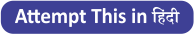Latest Banking jobs   »   Basic Reasoning Quiz for All Banking...

# Basic Reasoning Quiz for All Banking Exams- 1st June

Direction (1-5): Study the following information and answer the given question below:

9 7 2 9 9 4 8 7 7 2 7 5 9 4 8 2 9 4 6 5 7 1 4 3 2 4 1 6 6 3 9 8 2 2

Q1. How many such odd number are there in the above arrangement, each of which is immediately preceded by an even number and immediately followed by an odd number?
(a) None
(b) One
(c) Two
(d) Three
(e) More than three

Q2. How many such even numbers are there in the above arrangement each of which is immediately preceded by a prime number?
(a) None
(b) One
(c) Two
(d) Three
(e) More than three

Q3. Which of the following is the fifth to the right of the eight from the left end of the above arrangement?
(a) 8
(b) 9
(c) 4
(d) 5
(e) None of these

Q4. Which of the following is the fourth to the right of the twelfth from the right end of the above arrangement?
(a) 1
(b) 6
(c) 9
(d) 4
(e) 2

Q5.If all the prime numbers in the above arrangement are dropped, which of the following will be the sixth from the left end?
(a) 8
(b) 9
(c) 4
(d) 6
(e) None of these

Directions (6-10): These questions are based on the following five numbers:
245 674 978 887 635

Q6. If we arranged all numbers in ascending order from left end then, the position of how many numbers are remain unchanged?
(a) One
(b) None
(c) Three
(d) Two
(e) More than three

Q7. If we interchanged 1st and 3rd digit of each number within the number then, how many numbers thus formed are even numbers?
(a) None
(b) Three
(c) Two
(d) One
(e) More than three

Q8. If we interchanged 1st and 2nd digit of each number within the number then, which of the following number thus formed is 3rd smallest number?
(a) 245
(b) 674
(c) 978
(d) 887
(e) 635

Q9. If we arranged all digit in all numbers in descending order then, which of the following number thus formed is the smallest number?
(a) 245
(b) 674
(c) 978
(d) 887
(e) 635

Q10. What is the sum of 3rd digit of 2nd number from left end and 2nd digit of 3rd number from right end?
(a) 10
(b) 8
(c) 9
(d) 11
(e) None of these

Directions (11-15): The following questions are based on the five three digits number given below.
451 685 254 723 132
Q11. Which of the following numbers will be obtained if the second digit of the greatest number is subtracted from the second digit of the lowest number after adding one to each of the numbers?
(a) 1
(b) 2
(c) 3
(d) 4
(e) 5

Q12. If in each number, the first and the last digits are interchanged then, which of the following thus formed will be the third highest number?
(a) 451
(b) 685
(c) 254
(d) 723
(e) 132

Q13. If in each number, the second and the third digits are interchanged then, which will be the second highest number?
(a) 451
(b) 685
(c) 254
(d) 723
(e) 132

Q14. If one is subtracted from the first digit of each of the numbers and then the first and the third digits are interchanged, which of the following will be the lowest?
(a) 451
(b) 685
(c) 254
(d) 723
(e) 132

Q15. If in each number, all the three digits are arranged in the ascending order within the number, which of the following will be the third lowest number?
(a) 451
(b) 685
(c) 254
(d) 723
(e) 132

Practice More Questions of Reasoning for Competitive Exams:

SolutionsS1. Ans.(e)

S2. Ans.(e)

S3. Ans.(b)
Sol. fifth to the right of the eight from the left end of the above arrangement= 5+8=13th from left end= 9

S4. Ans.(a)
Sol. 4th to the right of the 12th from right means = 12-4= 8th from the right, i.e., 1.

S5. Ans.(b)
Sol. 9

S6. Ans.(d)

S7. Ans.(e)

S8. Ans.(b)

S9. Ans.(a)

S10. Ans.(d)

S11. Ans.(a)
Sol. 3-2=1

S12. Ans.(d)

S13. Ans.(b)

S14. Ans.(a)

S15. Ans.(d)